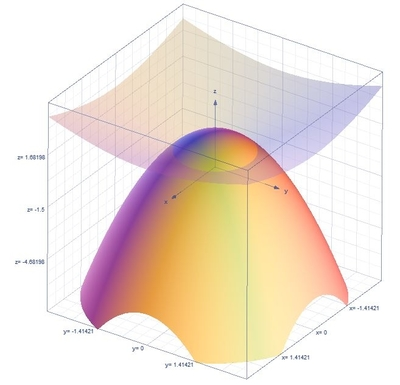# Find the volume of the "ice cream cone" formed by the cone z=\sqrt{x^2+y^2} and the paraboloid...

## Question:

Find the volume of the "ice cream cone" formed by the cone {eq}z=\sqrt{x^2+y^2}{/eq} and the paraboloid {eq}z=2-(x^2+y^2){/eq} by using a triple integral in cylindrical coordinate.

## Volume of the Region:

The triple integrals formula for the volume of the region in cylindrical coordinates is {eq}V=\int_{\alpha }^{\beta }\int_{h_{1}(\theta )}^{h_{2}(\theta )}\int_{u_{1}(r\cos \theta ,r\sin \theta )}^{u_{2}(r\cos \theta ,r\sin \theta )}r\:f\left ( r\cos \theta ,r\sin \theta ,z \right )dzdrd\theta {/eq} and the conversion formulas are the following {eq}r^{2}=x^{2}+y^{2},\:x=r\cos \theta ,\:y=r\sin \theta {/eq}.

## Answer and Explanation:

Below is the graph,Let us first find the limits, substituting one equation to the other we get

{eq}z=2-z^{2} {/eq}

{eq}z^{2}+z-2=0 {/eq}

{eq}\left ( z-1 \right )\left ( z+2 \right ) {/eq}

{eq}z=-2,1 {/eq}

We discard -2 because because based on the graph {eq}z {/eq} is above the xy plane. Thus {eq}z=1 {/eq}

Thus, the limits of integration are the following {eq}0\leq \theta \leq 2\theta ,\:0\leq r\leq 1,\:r\leq z\leq 2-r^{2} {/eq}

The volume of the region is,

{eq}V=\int_{0}^{2\pi }\int_{0}^{1}\int_{0}^{2-r^{2}}rdzdrd\theta {/eq}

Integrate with respect to {eq}z {/eq}

{eq}V=\int_{0}^{2\pi }\int_{0}^{1}\left [ z \right ]^{2-r^{2}}_{r}rdrd\theta {/eq}

{eq}V=\int_{0}^{2\pi }\int_{0}^{1}\left ( 2-r^{2}-r \right )rdrd\theta {/eq}

Integrate with respect to {eq}r {/eq}

{eq}V=\int_{0}^{2\pi }\left [ r^2-\frac{r^4}{4}-\frac{r^3}{3} \right ]^{1}_{0}d\theta {/eq}

{eq}V=\int_{0}^{2\pi }\frac{5}{12}d\theta {/eq}

Integrate with respect to {eq}\theta {/eq}

{eq}V=\frac{5}{12}\left [ \theta \right ]^{2\theta }_{0} {/eq}

{eq}V=\frac{5\pi }{6} {/eq}

#### Learn more about this topic:Volumes of Shapes: Definition & Examples

from GMAT Prep: Tutoring Solution

Chapter 11 / Lesson 9
45K